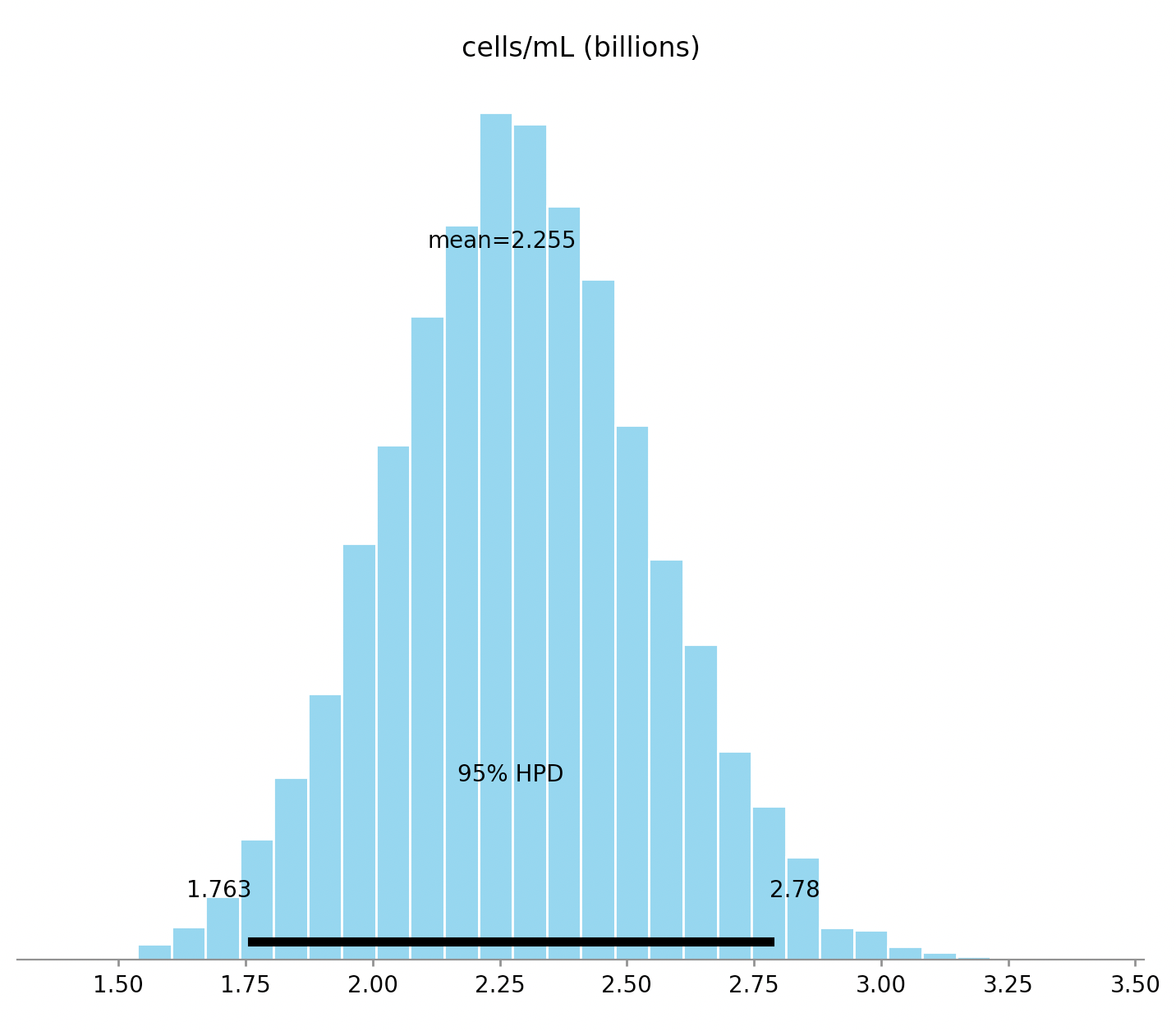# Bayesian cell counting

Posted by Cameron Davidson-Pilon on

Let’s say you are interested in counting the concentration of cells in some sample. This is a pretty common task: sperm counts, blood cell counts, plankton counts. Microbiologists are always counting. Let’s use the example of yeast counting, which is traditional in beer and wine making. The brewery has a sample of yeast slurry, a highly concentrated amount of yeast, and they would like to know how concentrated it is, so they can add the correct amount to a batch.

The first step is to dilute your sample. The original concentration could be as high as billions per mL, so even a fraction of a mL is going to be still too concentrated to properly count. This is solved by successive dilutions of the original sample:Each time we transfer to a new test tube, we dilute the sample by a factor of 10.

After this is done, a very small amount of the final diluted slurry is added to a hemocytometer. A hecocytometer is a glass slide with a visible grid that an observer can place under a microscope and count cells on. Under the microscope, it looks like this (where yellow dots represent cells):The hemocytometer is designed such that a known quantity of volume exists under the inner 25 squares (usually 0.0001 mL). The observer will apriori pick 5 squares to count cells in, typically the 4 corner squares and the middle square. (Why only 5? Unlike this image above, there could be thousands of cells, and counting all 25 squares would take too long). Since we know the volume of the 25 squares, and the dilution rate, we can recover our original slurry concentration:

$$\text{cells/mL} = (\text{cells counted}) \cdot 5 \cdot (\text{dilution factor}) / 0.0001 \text{mL}$$

Given I counted 49 yeast and my dilution was 1000x, my estimate is 2.45B yeast/mL. Great! We are done, right? No way, consider all the sources of uncertainty we glossed over:

1. Did we accurately measure out exactly 9mL of water in each test tube?
2. Did we accurately extract 1mL of slurry between each test tube?
3. Did we get lucky/unlucky with our 0.0001 mL sample for the hemocytometer?
4. Did the hemocytometer manufacturer have some QA over the volume of the chamber?
5. Did we get lucky/unlucky with cells numbers in the 5 counting squares?

So we should expect high variance in our estimate because of the many sources of noise, and because they are layered on top of one another. Let’s redo this with Bayesian statistics so we can model our uncertainty.

Here’s the code for an observation of 49 yeast cells counted. Each source of noise is a random variable. I’ve added some priors that I think are sensible.

import pymc3 as pm

BILLION = 1e9
TOTAL_SQUARES = 25

squares_counted = 5
yeast_counted = 49

with pm.Model() as model:
yeast_conc = pm.Normal("cells/mL", mu=2 * BILLION, sd=0.4 * BILLION)

shaker1_volume = pm.Normal("shaker1 volume (mL)", mu=9.0, sd=0.05)
shaker2_volume = pm.Normal("shaker2 volume (mL)", mu=9.0, sd=0.05)
shaker3_volume = pm.Normal("shaker3 volume (mL)", mu=9.0, sd=0.05)

yeast_slurry_volume = pm.Normal("initial yeast slurry volume (mL)", mu=1.0, sd=0.01)
shaker1_to_shaker2_volume =    pm.Normal("shaker1 to shaker2 (mL)", mu=1.0, sd=0.01)
shaker2_to_shaker3_volume =    pm.Normal("shaker2 to shaker3 (mL)", mu=1.0, sd=0.01)

dilution_shaker1 = yeast_slurry_volume       / (yeast_slurry_volume + shaker1_volume)
dilution_shaker2 = shaker1_to_shaker2_volume / (shaker1_to_shaker2_volume + shaker2_volume)
dilution_shaker3 = shaker2_to_shaker3_volume / (shaker2_to_shaker3_volume + shaker3_volume)
final_dilution_factor = dilution_shaker1 * dilution_shaker2 * dilution_shaker3

volume_of_chamber = pm.Gamma("volume of chamber (mL)", mu=0.0001, sd=0.0001 / 20)

# why is Poisson justified? in my final shaker, I have yeast_conc * final_dilution_factor * shaker3_volume number of yeast
# I remove volume_of_chamber / shaker3_volume fraction of them, hence it's a binomial with very high count, and very low probability.
yeast_visible = pm.Poisson("cells in visible portion", mu=yeast_conc * final_dilution_factor * volume_of_chamber)

number_of_counted_cells = pm.Binomial("number of counted cells", yeast_visible, squares_counted/TOTAL_SQUARES, observed=yeast_counted)

trace = pm.sample(5000, tune=1000)

pm.plot_posterior(trace, varnames=['cells/mL'])

The posterior for cells/mL is below:We can see that the width of the credible interval is about a billion. That’s surprisingly large. And that makes sense: we only have a single, noisy observation. We can see the influence of the prior here as well. Note that the posterior’s mean is about 2.25B, much closer to the priors’ mean of 2B than our naive estimate of 2.45B above. This brings up another point: using the naive formula above is like saying: “I observed a single coin flip, saw heads, so all coin flips are heads.” Sounds silly, but it’s identical inference. With Bayesian statistics, we get to lie in a much more reassuring bed!

Read the next article in this series, modelling growth